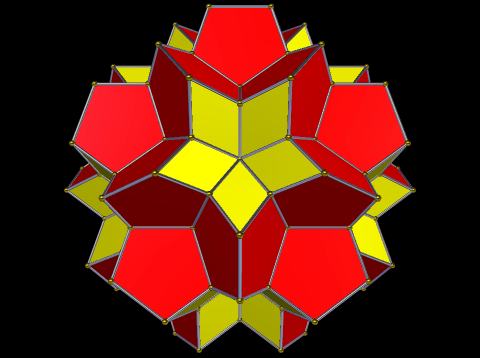Acronym ... Name p60-r60,41st stellation of ti© Vertex figure [r,52], [R2,52], [r5],  Dihedral angles between {5} and {5} at type 2 edges:   360°-arccos(-1/sqrt(5)) = 243.434949° between {(r,R)2} and {(r,R)2}:   360°-arccos(-sqrt(5)/3) = 221.810315° between {(r,R)2} and {5}:   arccos(-sqrt[(5-2 sqrt(5))/15]) = 100.812317° between {5} and {5} at type 1 edges:   arccos(1/sqrt(5)) = 63.434949°

The rhombs {(r,R)2} have vertex angles r = 60° resp. R = 120°. Esp. rr : RR = sqrt(3). – Alternatively the rhombs could be devided into pairs of coplanar regular triangles, but then asking for an additional edge each. – Accordingly, when filling the rhombic dimples halfway with peppies, this polyhedron could be transformed into a non-intersectingly concave polyhedron xfo3foo5oxf&#zx with regular polygons only.

This concave polyhedron also allows for an expansion (xfoa3fooo5xuFx&#zx), which then still is non-intersectingly concave, but where all faces then would be regular polygons. Thereby the rhombs will become hexagons.

Incidence matrix according to Dynkin symbol

xfoa3fooo5oxfo&#zx   → height = 0
a = sqrt(5) = 2.236068
(tegum sum of (x,f)-ti, (f,x)-srid, f-doe, and a-ike)

o...3o...5o...     | 60  *  *  * |  1   2  0  0 |  2  1  [r,52]
.o..3.o..5.o..     |  * 60  *  * |  0   2  1  1 |  2  2  [R2,52]
..o.3..o.5..o.     |  *  * 20  * |  0   0  3  0 |  3  0  
...o3...o5...o     |  *  *  * 12 |  0   0  0  5 |  0  5  [r5]
-------------------+-------------+--------------+------
x... .... ....     |  2  0  0  0 | 30   *  *  * |  2  0  (type 1)
oo..3oo..5oo..&#x  |  1  1  0  0 |  * 120  *  * |  1  1
.oo.3.oo.5.oo.&#x  |  0  1  1  0 |  *   * 60  * |  2  0  (type 2)
.o.o3.o.o5.o.o&#x  |  0  1  0  1 |  *   *  * 60 |  0  2
-------------------+-------------+--------------+------
xfo. .... ....&#xt |  2  2  1  0 |  1   2  2  0 | 60  *  {5}
.... .... ox.o&#xt |  1  2  0  1 |  0   2  0  2 |  * 60  {(r,R)2}